##### Print Instructions

NOTE: Only your test content will print.
To preview this test, click on the File menu and select Print Preview.

See our guide on How To Change Browser Print Settings to customize headers and footers before printing.

# Python - Basic Operators (Continuing Education)

Print Test (Only the test content will print)

## Python - Basic Operators

1.
Which of the following are constructs?
1. Symbols
2. Operators
3. Operations
4. Numerators
2.
Operators can manipulate the value of
1. operands.
2. numerators.
3. comparators.
4. denominators.
3.
Which of the following are types of operators? Check all that apply.
1. Bitwise
2. Symbolic
3. Arithmetic
4. Assignment
4.
What will the following lines of code produce?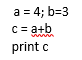1. 1
2. 3
3. 7
4. -1
5.
What will the following lines of code produce?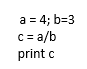1. 0
2. 1
3. 0.75
4. 1.3333
6.
What will the following code produce?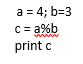1. -1
2. 1
3. 3
4. 4
7.
What will the following code produce?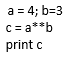1. 1
2. 12
3. 64
4. 81
8.
Which of the following is not a comparison operator?
1. =
2. >=
3. ==
4. !=
9.
Which value of ‘a’ would return “This is true” from the following code?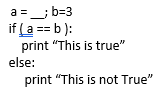1. 2
2. 3
3. 3.0
4. 5
10.
Which value of ‘a’ would not return “This is true” from the following code?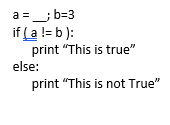1. 3
2. 3.0
3. 8
4. 10
11.
Which value of ‘a would return “This is true” from the following code?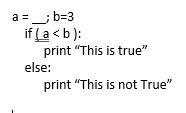1. 2
2. 3
3. 8
4. 10
12.
What will the following code produce?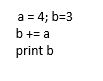1. 1
2. 3
3. 4
4. 7
13.
What will the following code produce?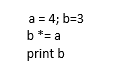1. 1
2. 4
3. 12
4. 64
14.
If a = 0110 0011, and b = 0101 0010, then a&b =
1. 0011 0001.
2. 0100 0010.
3. 0011 0010.
4. 0111 0011.
15.
If a = 0110 0011, and b = 0101 0010, then a|b =
1. 0011 0001.
2. 0100 0010.
3. 0111 0011.
4. 0011 0011.
16.
If a = 0110 0011, and b = 0101 0010, then a^b =
1. 0011 0001.
2. 0100 0010.
3. 0111 0011.
4. 0111 0100.
You need to be a HelpTeaching.com member to access free printables.